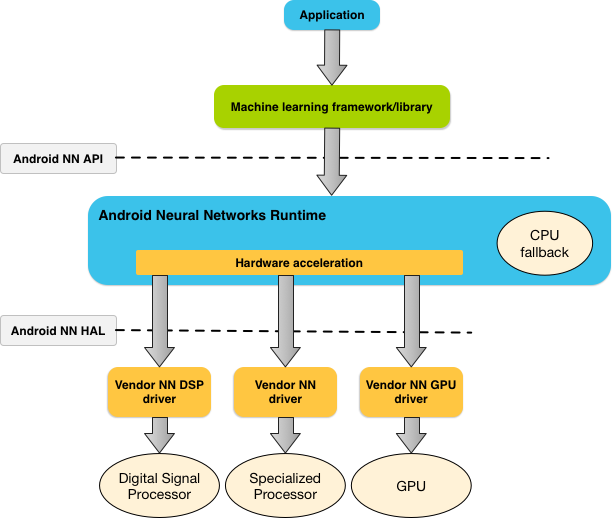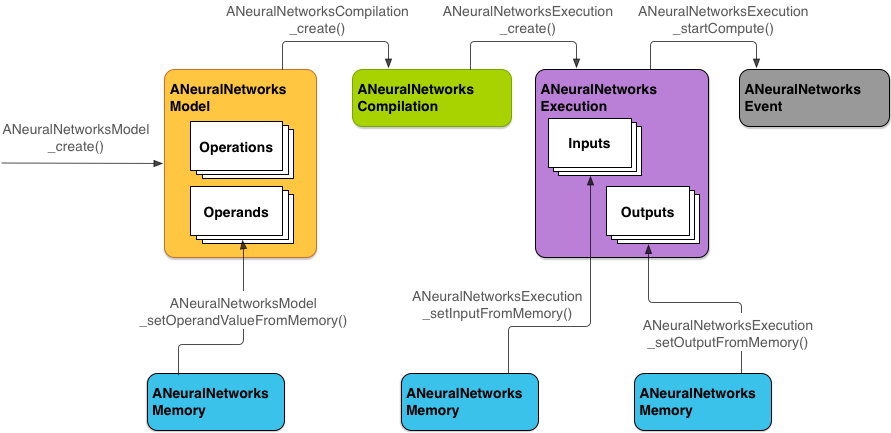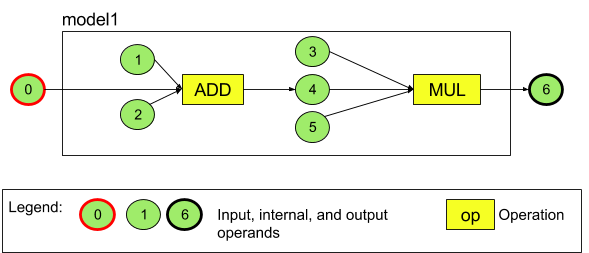# Neural Networks API

The Android Neural Networks API (NNAPI) is an Android C API designed for running computationally intensive operations for machine learning on mobile devices. NNAPI is designed to provide a base layer of functionality for higher-level machine learning frameworks (such as TensorFlow Lite, Caffe2, or others) that build and train neural networks. The API is available on all devices running Android 8.1 (API level 27) or higher.

NNAPI supports inferencing by applying data from Android devices to previously trained, developer-defined models. Examples of inferencing include classifying images, predicting user behavior, and selecting appropriate responses to a search query.

On-device inferencing has many benefits:

• Latency: You don’t need to send a request over a network connection and wait for a response. This can be critical for video applications that process successive frames coming from a camera.
• Availability: The application runs even when outside of network coverage.
• Speed: New hardware specific to neural networks processing provide significantly faster computation than with general-use CPU alone.
• Privacy: The data does not leave the device.
• Cost: No server farm is needed when all the computations are performed on the device.

There are also trade-offs that a developer should keep in mind:

• System utilization: Evaluating neural networks involve a lot of computation, which could increase battery power usage. You should consider monitoring the battery health if this is a concern for your app, especially for long-running computations.
• Application size: Pay attention to the size of your models. Models may take up multiple megabytes of space. If bundling large models in your APK would unduly impact your users, you may want to consider downloading the models after app installation, using smaller models, or running your computations in the cloud. NNAPI does not provide functionality for running models in the cloud.

Related sample:

## Understanding the Neural Networks API runtime

NNAPI is meant to be called by machine learning libraries, frameworks, and tools that let developers train their models off-device and deploy them on Android devices. Apps typically would not use NNAPI directly, but would instead directly use higher-level machine learning frameworks. These frameworks in turn could use NNAPI to perform hardware-accelerated inference operations on supported devices.

Based on the app’s requirements and the hardware capabilities on a device, Android’s neural networks runtime can efficiently distribute the computation workload across available on-device processors, including dedicated neural network hardware, graphics processing units (GPUs), and digital signal processors (DSPs).

For devices that lack a specialized vendor driver, the NNAPI runtime relies on optimized code to execute requests on the CPU.

Figure 1 shows a high-level system architecture for NNAPI.Figure 1. System architecture for Android Neural Networks API

## Neural Networks API programming model

To perform computations using NNAPI, you first need to construct a directed graph that defines the computations to perform. This computation graph, combined with your input data (for example, the weights and biases passed down from a machine learning framework), forms the model for NNAPI runtime evaluation.

NNAPI uses four main abstractions:

• Model: A computation graph of mathematical operations and the constant values learned through a training process. These operations are specific to neural networks. They include 2-dimensional (2D) convolution, logistic (sigmoid) activation, rectified linear (ReLU) activation, and more. Creating a model is a synchronous operation, but once successfully created, it can be reused across threads and compilations. In NNAPI, a model is represented as an `ANeuralNetworksModel` instance.
• Compilation: Represents a configuration for compiling an NNAPI model into lower-level code. Creating a compilation is a synchronous operation, but once successfully created, it can be reused across threads and executions. In NNAPI, each compilation is represented as an `ANeuralNetworksCompilation` instance.
• Memory: Represents shared memory, memory mapped files, and similar memory buffers. Using a memory buffer lets the NNAPI runtime transfer data to drivers more efficiently. An app typically creates one shared memory buffer that contains every tensor needed to define a model. You can also use memory buffers to store the inputs and outputs for an execution instance. In NNAPI, each memory buffer is represented as an `ANeuralNetworksMemory` instance.
• Execution: Interface for applying an NNAPI model to a set of inputs and to gather the results. Execution is an asynchronous operation. Multiple threads can wait on the same execution. When the execution completes, all threads will be released. In NNAPI, each execution is represented as an `ANeuralNetworksExecution` instance.

Figure 2 shows the basic programming flow.Figure 2. Programming flow for Android Neural Networks API

The rest of this section describes the steps to set up your NNAPI model to perform computation, compile the model, and execute the compiled model.

### Providing access to training data

Your trained weights and biases data are likely stored in a file. To provide the NNAPI runtime with efficient access to this data, create a `ANeuralNetworksMemory` instance by calling the `ANeuralNetworksMemory_createFromFd()` function, and passing in the file descriptor of the opened data file.

You can also specify memory protection flags and an offset where the shared memory region starts in the file.

``````// Create a memory buffer from the file that contains the trained data.
ANeuralNetworksMemory* mem1 = NULL;
int fd = open("training_data", O_RDONLY);
ANeuralNetworksMemory_createFromFd(file_size, PROT_READ, fd, 0, &mem1);
``````

Although in this example we use only one `ANeuralNetworksMemory` instance for all our weights, it’s possible to use more than one `ANeuralNetworksMemory` instance for multiple files.

### Models

A model is the fundamental unit of computation in NNAPI. Each model is defined by one or more operands and operations.

#### Operands

Operands are data objects used in defining the graph. These include the inputs and outputs of the model, the intermediate nodes that contain the data that flows from one operation to the other, and the constants that are passed to these operations.

There are two types of operands that can be added to NNAPI models: scalars and tensors.

A scalar represents a single number. NNAPI supports scalar values in 32-bit floating point, 32-bit integer, and unsigned 32-bit integer format.

Most operations with NNAPI involve tensors. Tensors are n-dimensional arrays. NNAPI supports tensors with 32-bit integer, 32-bit floating point, and 8-bit quantized values.

For example, figure 3 represents a model with two operations: an addition followed by a multiplication. The model takes an input tensor and produces one output tensor.Figure 3. Example of operands for an NNAPI model

The model above has seven operands. These operands are identified implicitly by the index of the order in which they are added to the model. The first operand added has an index of 0, the second an index of 1, and so on.

The order in which you add the operands does not matter. For example, the model output operand could be the first one added. The important part is to use the right index value when referring to an operand.

Operands have types. These are specified when they are added to the model. An operand cannot be used as both input and output of a model.

For additional topics on using operands, see More about operands.

#### Operations

An operation specifies the computations to be performed. Each operation consists of these elements:

• an operation type (for example, addition, multiplication, convolution),
• a list of indexes of the operands that the operation uses for input, and
• a list of indexes of the operands that the operation uses for output.

The order in these lists matters; see the NNAPI API reference for each operation for the expected inputs and outputs.

You must add the operands that an operation consumes or produces to the model before the adding the operation.

The order in which you add operations does not matter. NNAPI relies on the dependencies established by the computation graph of operands and operations to determine the order in which operations are executed.

The operations that NNAPI supports are summarized in the table below:

Category Operations
Element-wise mathematical operations
• `ANEURALNETWORKS_ADD`
• `ANEURALNETWORKS_MUL`
• `ANEURALNETWORKS_DIV`
• `ANEURALNETWORKS_SUB`
• `ANEURALNETWORKS_FLOOR`
Array operations
• `ANEURALNETWORKS_CONCATENATION`
• `ANEURALNETWORKS_DEQUANTIZE`
• `ANEURALNETWORKS_RESHAPE`
• `ANEURALNETWORKS_SQUEEZE`
• `ANEURALNETWORKS_TRANSPOSE`
• `ANEURALNETWORKS_PAD`
• `ANEURALNETWORKS_MEAN`
• `ANEURALNETWORKS_STRIDED_SLICE`
• `ANEURALNETWORKS_SPACE_TO_DEPTH`
• `ANEURALNETWORKS_DEPTH_TO_SPACE`
• `ANEURALNETWORKS_SPACE_TO_BATCH_ND`
• `ANEURALNETWORKS_BATCH_TO_SPACE_ND`
Image operations
• `ANEURALNETWORKS_RESIZE_BILINEAR`
Lookup operations
• `ANEURALNETWORKS_HASHTABLE_LOOKUP`
• `ANEURALNETWORKS_EMBEDDING_LOOKUP`
Normalization operations
• `ANEURALNETWORKS_L2_NORMALIZATION`
• `ANEURALNETWORKS_LOCAL_RESPONSE_NORMALIZATION`
Convolution operations
• `ANEURALNETWORKS_CONV_2D`
• `ANEURALNETWORKS_DEPTHWISE_CONV_2D`
Pooling operations
• `ANEURALNETWORKS_AVERAGE_POOL_2D`
• `ANEURALNETWORKS_L2_POOL_2D`
• `ANEURALNETWORKS_MAX_POOL_2D`
Activation operations
• `ANEURALNETWORKS_LOGISTIC`
• `ANEURALNETWORKS_RELU`
• `ANEURALNETWORKS_RELU1`
• `ANEURALNETWORKS_RELU6`
• `ANEURALNETWORKS_SOFTMAX`
• `ANEURALNETWORKS_TANH`
Other operations
• `ANEURALNETWORKS_FULLY_CONNECTED`
• `ANEURALNETWORKS_LSH_PROJECTION`
• `ANEURALNETWORKS_LSTM`
• `ANEURALNETWORKS_RNN`
• `ANEURALNETWORKS_SVDF`

Known issue: When passing `ANEURALNETWORKS_TENSOR_QUANT8_ASYMM` tensors to the `ANEURALNETWORKS_PAD` operation, which is available on Android 9 (API level 28) and higher, the output from NNAPI may not match output from higher-level machine learning frameworks, such as TensorFlow Lite. You should instead pass only `ANEURALNETWORKS_TENSOR_FLOAT32` until the issue is resolved.

#### Building models

To build a model, follow these steps:

1. Call the `ANeuralNetworksModel_create()` function to define an empty model.

In the following example, we create the two-operation model found in figure 3.

``````ANeuralNetworksModel* model = NULL;
ANeuralNetworksModel_create(&model);
``````
2. Add the operands to your model by calling `ANeuralNetworks_addOperand()`. Their data types are defined using the `ANeuralNetworksOperandType` data structure.

``````// In our example, all our tensors are matrices of dimension .
ANeuralNetworksOperandType tensor3x4Type;
tensor3x4Type.type = ANEURALNETWORKS_TENSOR_FLOAT32;
tensor3x4Type.scale = 0.f;    // These fields are useful for quantized tensors.
tensor3x4Type.zeroPoint = 0;  // These fields are useful for quantized tensors.
tensor3x4Type.dimensionCount = 2;
uint32_t dims = {3, 4};
tensor3x4Type.dimensions = dims;

// We also specify operands that are activation function specifiers.
ANeuralNetworksOperandType activationType;
activationType.type = ANEURALNETWORKS_INT32;
activationType.scale = 0.f;
activationType.zeroPoint = 0;
activationType.dimensionCount = 0;
activationType.dimensions = NULL;

// Now we add the seven operands, in the same order defined in the diagram.
ANeuralNetworksModel_addOperand(model, &tensor3x4Type);  // operand 0
ANeuralNetworksModel_addOperand(model, &tensor3x4Type);  // operand 1
ANeuralNetworksModel_addOperand(model, &activationType); // operand 2
ANeuralNetworksModel_addOperand(model, &tensor3x4Type);  // operand 3
ANeuralNetworksModel_addOperand(model, &tensor3x4Type);  // operand 4
ANeuralNetworksModel_addOperand(model, &activationType); // operand 5
ANeuralNetworksModel_addOperand(model, &tensor3x4Type);  // operand 6
``````
3. For operands that have constant values, such as weights and biases that your app obtains from a training process, use the `ANeuralNetworksModel_setOperandValue()` and `ANeuralNetworksModel_setOperandValueFromMemory()` functions.

In the following example, we set constant values from the training data file for which we created the memory buffer above.

``````// In our example, operands 1 and 3 are constant tensors whose value was
// established during the training process.
const int sizeOfTensor = 3 * 4 * 4;    // The formula for size calculation is dim0 * dim1 * elementSize.
ANeuralNetworksModel_setOperandValueFromMemory(model, 1, mem1, 0, sizeOfTensor);
ANeuralNetworksModel_setOperandValueFromMemory(model, 3, mem1, sizeOfTensor, sizeOfTensor);

// We set the values of the activation operands, in our example operands 2 and 5.
int32_t noneValue = ANEURALNETWORKS_FUSED_NONE;
ANeuralNetworksModel_setOperandValue(model, 2, &noneValue, sizeof(noneValue));
ANeuralNetworksModel_setOperandValue(model, 5, &noneValue, sizeof(noneValue));
``````
4. For each operation in the directed graph you want to compute, add the operation to your model by calling the `ANeuralNetworksModel_addOperation()` function.

As parameters to this call, your app must provide:

• the operation type,
• the count of input values,
• the array of the indexes for input operands,
• the count of output values, and
• the array of the indexes for output operands.

Note that an operand cannot be used for both input and output of the same operation.

``````// We have two operations in our example.
// The first consumes operands 1, 0, 2, and produces operand 4.
uint32_t addInputIndexes = {1, 0, 2};
uint32_t addOutputIndexes = {4};
ANeuralNetworksModel_addOperation(model, ANEURALNETWORKS_ADD, 3, addInputIndexes, 1, addOutputIndexes);

// The second consumes operands 3, 4, 5, and produces operand 6.
uint32_t multInputIndexes = {3, 4, 5};
uint32_t multOutputIndexes = {6};
ANeuralNetworksModel_addOperation(model, ANEURALNETWORKS_MUL, 3, multInputIndexes, 1, multOutputIndexes);
``````
5. Identify which operands the model should treat as its inputs and outputs, by calling the `ANeuralNetworksModel_identifyInputsAndOutputs()` function. This function lets you configure the model to use a subset of the input and output operands that you specified earlier in step 4.

``````// Our model has one input (0) and one output (6).
uint32_t modelInputIndexes = {0};
uint32_t modelOutputIndexes = {6};
ANeuralNetworksModel_identifyInputsAndOutputs(model, 1, modelInputIndexes, 1 modelOutputIndexes);
``````
6. Optionally, specify whether `ANEURALNETWORKS_TENSOR_FLOAT32` is allowed to be calculated with range or precision as low as that of the IEEE 754 16-bit floating-point format by calling `ANeuralNetworksModel_relaxComputationFloat32toFloat16()`.

7. Call `ANeuralNetworksModel_finish()` to finalize the definition of your model. If there are no errors, this function returns a result code of `ANEURALNETWORKS_NO_ERROR`.

``````ANeuralNetworksModel_finish(model);
``````

Once you create a model, you can compile it any number of times and execute each compilation any number of times.

## Compilation

The compilation step determines on which processors your model will be executed and asks the corresponding drivers to prepare for its execution. This could include the generation of machine code specific to the processors on which your model will run.

To compile a model, follow these steps:

1. Call the `ANeuralNetworksCompilation_create()` function to create a new compilation instance.

``````// Compile the model.
ANeuralNetworksCompilation* compilation;
ANeuralNetworksCompilation_create(model, &compilation);
``````
2. You can optionally influence how the runtime trades off between battery power usage and execution speed. You can do so by calling `ANeuralNetworksCompilation_setPreference()`.

``````// Ask to optimize for low power consumption.
ANeuralNetworksCompilation_setPreference(compilation, ANEURALNETWORKS_PREFER_LOW_POWER);
``````

The valid preferences you can specify include:

3. Finalize the compilation definition by calling `ANeuralNetworksCompilation_finish()`. If there are no errors, this function returns a result code of `ANEURALNETWORKS_NO_ERROR`.

``````ANeuralNetworksCompilation_finish(compilation);
``````

## Execution

The execution step applies the model to a set of inputs, and stores the computation outputs to one or more user buffers or memory spaces that your app allocated.

To execute a compiled model, follow these steps:

1. Call the `ANeuralNetworksExecution_create()` function to create a new execution instance.

``````// Run the compiled model against a set of inputs.
ANeuralNetworksExecution* run1 = NULL;
ANeuralNetworksExecution_create(compilation, &run1);
``````
2. Specify where your app reads the input values for the computation. Your app can read input values from either a user buffer or an allocated memory space, by calling `ANeuralNetworksExecution_setInput()` or `ANeuralNetworksExecution_setInputFromMemory()` respectively.

``````// Set the single input to our sample model. Since it is small, we won’t use a memory buffer.
float32 myInput = { ..the data.. };
ANeuralNetworksExecution_setInput(run1, 0, NULL, myInput, sizeof(myInput));
``````
3. Specify where your app writes the output values. Your app can write output values to either a user buffer or an allocated memory space, by calling `ANeuralNetworksExecution_setOutput()` or `ANeuralNetworksExecution_setOutputFromMemory()` respectively.

``````// Set the output.
float32 myOutput;
ANeuralNetworksExecution_setOutput(run1, 0, NULL, myOutput, sizeof(myOutput));
``````
4. Schedule the execution to start, by calling the `ANeuralNetworksExecution_startCompute()` function. If there are no errors, this function returns a result code of `ANEURALNETWORKS_NO_ERROR`.

``````// Starts the work. The work proceeds asynchronously.
ANeuralNetworksEvent* run1_end = NULL;
ANeuralNetworksExecution_startCompute(run1, &run1_end);
``````
5. Call the `ANeuralNetworksEvent_wait()` function to wait for the execution to complete. If the execution was successful, this function returns a result code of `ANEURALNETWORKS_NO_ERROR`. Waiting can be done on a different thread than the one starting the execution.

``````// For our example, we have no other work to do and will just wait for the completion.
ANeuralNetworksEvent_wait(run1_end);
ANeuralNetworksEvent_free(run1_end);
ANeuralNetworksExecution_free(run1);
``````
6. Optionally, you can apply a different set of inputs to the compiled model by using the same compilation instance to create a new `ANeuralNetworksExecution` instance.

``````// Apply the compiled model to a different set of inputs.
ANeuralNetworksExecution* run2;
ANeuralNetworksExecution_create(compilation, &run2);
ANeuralNetworksExecution_setInput(run2, ...);
ANeuralNetworksExecution_setOutput(run2, ...);
ANeuralNetworksEvent* run2_end = NULL;
ANeuralNetworksExecution_startCompute(run2, &run2_end);
ANeuralNetworksEvent_wait(run2_end);
ANeuralNetworksEvent_free(run2_end);
ANeuralNetworksExecution_free(run2);
``````

## Cleanup

The cleanup step handles the freeing of internal resources used for your computation.

``````// Cleanup
ANeuralNetworksCompilation_free(compilation);
ANeuralNetworksModel_free(model);
ANeuralNetworksMemory_free(mem1);
``````

## More about operands

The following section covers advanced topics about using operands.

### Quantized tensors

A quantized tensor is a compact way to represent an n-dimensional array of floating point values.

NNAPI supports 8-bit asymmetric quantized tensors. For these tensors, the value of each cell is represented by an 8-bit integer. Associated with the tensor is a scale and a zero point value. These are used to convert the 8-bit integers into the floating point values that are being represented.

The formula is:

``````(cellValue - zeroPoint) * scale
``````

where the zeroPoint value is a 32-bit integer and the scale a 32-bit floating point value.

Compared to tensors of 32-bit floating point values, 8-bit quantized tensors have two advantages:

• Your application will be smaller, as the trained weights will take a quarter of the size of 32-bit tensors.
• Computations can often be executed faster. This is due to the smaller amount of data that needs to be fetched from memory and the efficiency of processors such as DSPs in doing integer math.

While it is possible to convert a floating point model to a quantized one, our experience has shown that better results are achieved by training a quantized model directly. In effect, the neural network learns to compensate for the increased granularity of each value. For each quantized tensor, the scale and zeroPoint values are determined during the training process.

In NNAPI, you define quantized tensors types by setting the type field of the `ANeuralNetworksOperandType` data structure to `ANEURALNETWORKS_TENSOR_QUANT8_ASYMM`. You also specify the scale and zeroPoint value of the tensor in that data structure.

### Optional operands

A few operations, like `ANEURALNETWORKS_LSH_PROJECTION`, take optional operands. To indicate in the model that the optional operand is omitted, call the `ANeuralNetworksModel_setOperandValue()` function, passing `NULL` for the buffer and 0 for the length.

If the decision on whether the operand is present or not varies for each execution, you indicate that the operand is omitted by using the `ANeuralNetworksExecution_setInput()` or `ANeuralNetworksExecution_setOutput()` functions, passing `NULL` for the buffer and 0 for the length.